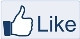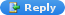# IMAGE PROCESSING

" Two roads diverged in a wood, and I,
I took the one less traveled by,
And that has made all the difference "-Robert Frost

### Gaussian Filter without using the MATLAB built_in function

Gaussian Filter

Gaussian Filter is used to blur the image. It is used to reduce the noise and the image details.

The Gaussian kernel's center part  ( Here 0.4421 )  has the highest value and  intensity of other pixels  decrease as the distance from the center part increases.

On convolution of the local region and the Gaussian kernel gives the highest intensity value to the center part of the local region(38.4624) and the remaining pixels have less intensity as the distance from the center increases.
Sum up the result and store it in the current pixel location(Intensity = 94.9269) of the image.

MATLAB CODE:
%Gaussian filter using MATLAB built_in function
A = imnoise(Img,'Gaussian',0.04,0.003);
%Image with noise
figure,imshow(A);

H = fspecial('Gaussian',[9 9],1.76);
GaussF = imfilter(A,H);
figure,imshow(GaussF);

MATLAB CODE for Gaussian blur WITHOUT built_in function:
A = imnoise(Img,'Gaussian',0.04,0.003);
%Image with noise
figure,imshow(A);
I = double(A);

%Design the Gaussian Kernel
%Standard Deviation
sigma = 1.76;
%Window size
sz = 4;
[x,y]=meshgrid(-sz:sz,-sz:sz);

M = size(x,1)-1;
N = size(y,1)-1;
Exp_comp = -(x.^2+y.^2)/(2*sigma*sigma);
Kernel= exp(Exp_comp)/(2*pi*sigma*sigma);Gaussian Kernel 9x9 size with Standard Deviation =1.76
%Initialize
Output=zeros(size(I));

%Convolution
for i = 1:size(I,1)-M
for j =1:size(I,2)-N
Temp = I(i:i+M,j:j+M).*Kernel;
Output(i,j)=sum(Temp(:));
end
end
%Image without Noise after Gaussian blur
Output = uint8(Output);
figure,imshow(Output);After FilteringLike "IMAGE PROCESSING" page

Unknown said...Geat job!! Thanks.!Anonymous said...How did you find Gaussian kernel kindly explain briefly

Unknown said...hi can you please send me mathematical equation for 3D images

yassir sall said...nice code i want to get the kernel how can i do that?all the reference are not downloading it

yassir sall said...nice code i want to get the kernel i don't have it and all the reference not useful can you give me an excellent one?

Unknown said...its not working

rock_hound said...How is the center pixel = 0 for the exp(-(x^2+y^2)/(2*sigma^2))? When x and y are both zero, the exponent = 0 and exp(0) = 1.

Aaron Angel said...@rock_hound

The explanation is for -x^2+y^2)/(2*sigma*sigma).
Thank you

Unknown said...Very Interesting code !! please i want to get the kernel i don't have it and all the reference not useful can you give me an excellent one?

Unknown said...This is not working.

sumanayana said...we need a correct one line matlab command using gaussian filter to remove noise

catherine said...hi, can you explain me the different between denoising image with gaussian filter in spatial domain and frequency domain? how to differentiate it with your code?